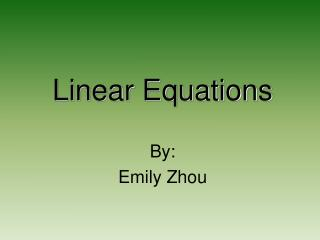DownloadDownload PresentationLinear Equations

# Linear Equations

Télécharger la présentation## Linear Equations

- - - - - - - - - - - - - - - - - - - - - - - - - - - E N D - - - - - - - - - - - - - - - - - - - - - - - - - - -
##### Presentation Transcript

1. Linear Equations By: Emily Zhou

2. What is a Linear Equation? • A linear equation is an equation whose graph forms a straight line. • Linear equations are usually shown on a coordinate plane • Real life situations of linear equations include the stock market as well as the payments of a car.

3. Parts of a Coordinate Plane QUADRANT II (-x, y) QUADRANT I (x, y) Origin QUADRANT III (-x, -y) QUADRANT IV (x, -y) X-Axis Y-Axis

4. Slope • Slope is the ratio of vertical change to the horizontal change (rise/run) of a line. • Slope in a linear equation shows if the line is ascending (positive) or descending (negative). • Slope can also show the rate of change. • The letter m is used to represent slope in a formula.

5. Forms of Linear Equations • The forms of linear equations are the formats in which the information is written in. • These two forms are the most commonly used ways to write linear equations. 1. Slope InterceptForm: y=mx+b 2. Standard Form: Ax + By =C

6. Slope Intercept Form • Slope intercept form is y=mx+b. • This form makes it easy to find the slope (m) and the y-intercept (b). • Working with this form is simple, so it is used more often than other forms. Example: y= ¾x + 3 *¾is the slope. * 3 is the point where the line crosses the Y-axis.

7. Graph Using Slope Intercept Form I will use the equation y= ½ x-1 to demonstrate how to graph a linear equation using slope intercept form. 1.)First, graph the y-intercept (b). In this equation, b= -1, so place a dot on the point (0,-1). 2.)Next, use the slope (½ ) to rise up once and run twice horizontally. Plot the point. 3.)Plot as many points as desired using the slope, then draw a line through the points. Run 2 Rise 1

8. Standard Form • Standard form for linear equations is Ax + By=C. • It can be used to find two points on the line of the equation. Example: 2x+2y=4 1.)Substitute in a zero for x. Simplify. 2(0)+2y=4 y=2 2.)One point of the line is (0,2). Plot the point. 3.) Substitute y with zero. Simplify. 2x+2(0)=4 x=2 4.)The second point is (2,0). Plot it and draw a line through the two points.

9. Using Linear Equations • Linear equations are not only a main part of algebra courses, but it is just as important in the real world. • If you deposited \$40 in the bank every year, and you would receive 7% each year, how much will you have in 15 years? Using a linear equation to graph it out, it would give you a better idea about what how much you will have in the future. • No matter what kind of situations, linear equations help to make life easier.